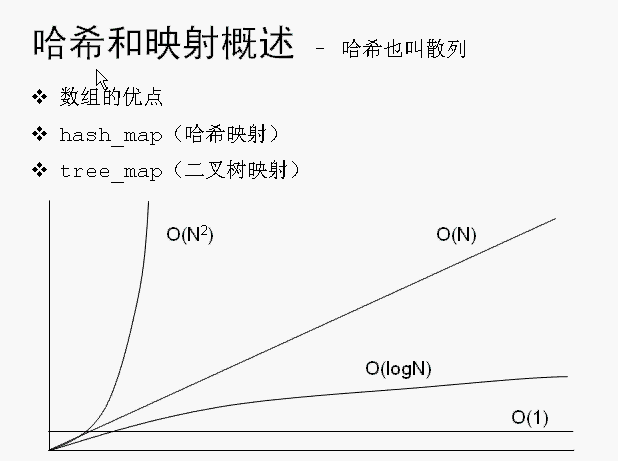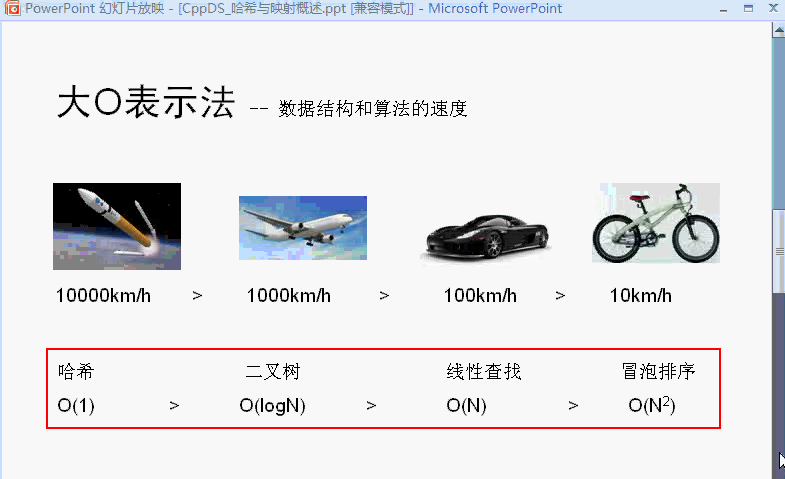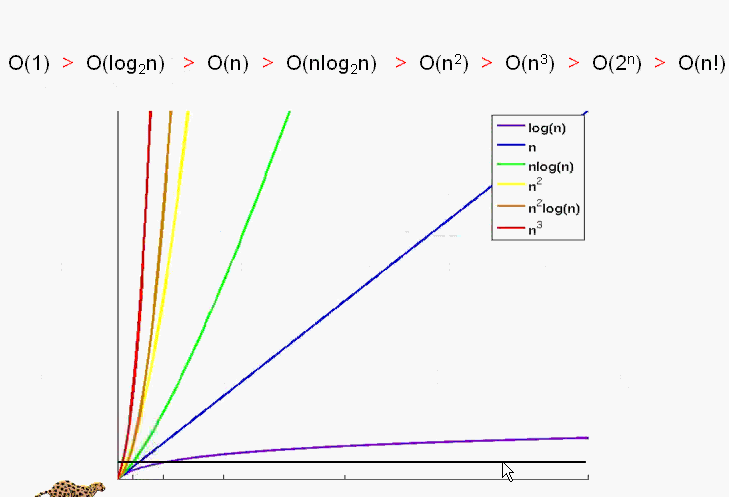## C++：Hash_map(哈希映射)__123杨子江的博客-程序员秘密## 0 为什么需要hash_map

``````岳不群－华山派掌门人，人称君子剑

...
``````

``````#include <map>
#include <string>
using namespace std;
...
map<string, string> namemap;
//增加。。。
namemap["岳不群"]="华山派掌门人，人称君子剑";
namemap["张三丰"]="武当掌门人，太极拳创始人";
namemap["东方不败"]="第一高手，葵花宝典";
...
//查找。。
if(namemap.find("岳不群") != namemap.end()){

...
}
``````

## 1 数据结构：hash_map原理

hash_map基于hash table（哈希表）。 哈希表最大的优点，就是把数据的存储和查找消耗的时间大大降低，几乎可以看成是常数时间；而代价仅仅是消耗比较多的内存。然而在当前可利用内存越来越多的情况下，用空间换时间的做法是值得的。另外，编码比较容易也是它的特点之一。

hash_map，首先分配一大片内存，形成许多桶。是利用hash函数，对key进行映射到不同区域（桶）进行保存。其插入过程是：

1. 得到key
2. 通过hash函数得到hash值
3. 得到桶号(一般都为hash值对桶数求模)
4. 存放key和value在桶内。

1. 得到key
2. 通过hash函数得到hash值
3. 得到桶号(一般都为hash值对桶数求模)
4. 比较桶的内部元素是否与key相等，若都不相等，则没有找到。
5. 取出相等的记录的value。

hash_map中直接地址用hash函数生成，解决冲突，用比较函数解决。这里可以看出，如果每个桶内部只有一个元素，那么查找的时候只有一次比较。当许多桶内没有值时，许多查询就会更快了(指查不到的时候).

## 2 hash_map 使用

### 2.1 一个简单实例

``````#include <hash_map>
#include <string>
using namespace std;
int main(){

hash_map<int, string> mymap;
mymap="唐伯虎点秋香";
mymap="百万富翁的生活";
mymap="白领的工资底线";
...
if(mymap.find(10000) != mymap.end()){

...
}
``````

``````template <class _Key, class _Tp, class _HashFcn = hash<_Key>,
class _EqualKey = equal_to<_Key>,
class _Alloc = __STL_DEFAULT_ALLOCATOR(_Tp) >
class hash_map
{

...
}
``````

``````...
hash_map<int, string> mymap;
//等同于:
hash_map<int, string, hash<int>, equal_to<int> > mymap;
``````

Alloc我们就不要取关注太多了(希望深入了解Allocator的朋友可以参看标准库 STL ：Allocator能做什么)

hash_map类在头文件hash_map中，和所有其它的C++标准库一样，头文件没有扩展名。如下声明：

``````1 #include <hash_map>
2 using namespace std;
3 using namespace stdext;
``````

hash_map是一个聚合类，它继承自_Hash类，包括一个vector，一个list和一个pair，其中vector用于保存桶，list用于进行冲突处理，pair用于保存key->value结构，简要地伪码如下：

``````1 class hash_map<class _Tkey, class _Tval>
2 {

3 private:
4     typedef pair<_Tkey, _Tval> hash_pair;
5     typedef list<hash_pair>    hash_list;
6     typedef vector<hash_list>  hash_table;
7 };
``````

### 2.2 hash_map 的hash函数

hash< int>到底是什么样子？看看源码:

``````struct hash<int> {

size_t operator()(int __x) const {
return __x; }
};
``````

``````struct hash<char*>
struct hash<const char*>
struct hash<char>
struct hash<unsigned char>
struct hash<signed char>
struct hash<short>
struct hash<unsigned short>
struct hash<int>
struct hash<unsigned int>
struct hash<long>
struct hash<unsigned long>
``````

``````struct str_hash{

size_t operator()(const string& str) const
{

unsigned long __h = 0;
for (size_t i = 0 ; i < str.size() ; i ++)
__h = 5*__h + str[i];
return size_t(__h);
}
};
//如果你希望利用系统定义的字符串hash函数，你可以这样写：
struct str_hash{

size_t operator()(const string& str) const
{

return __stl_hash_string(str.c_str());
}
};
``````

1. 使用struct，然后重载operator().
2. 返回是size_t
3. 参数是你要hash的key的类型。
4. 函数是const类型的。

``````map<string, string> namemap;
//改为：
hash_map<string, string, str_hash> namemap;
``````

### 2.3 hash_map 的比较函数

``````//本代码可以从SGI STL
//先看看binary_function 函数声明，其实只是定义一些类型而已。
template <class _Arg1, class _Arg2, class _Result>
struct binary_function {

typedef _Arg1 first_argument_type;
typedef _Arg2 second_argument_type;
typedef _Result result_type;
};
//看看equal_to的定义：
template <class _Tp>
struct equal_to : public binary_function<_Tp,_Tp,bool>
{

bool operator()(const _Tp& __x, const _Tp& __y) const {
return __x == __y; }
};
``````

``````struct mystruct{

int iID;
int  len;
bool operator==(const mystruct & my) const{

return (iID==my.iID) && (len==my.len) ;
}
};

struct compare_str{

bool operator()(const char* p1, const char*p2) const{

return strcmp(p1,p2)==0;
}
};

typedef hash_map<const char*, string, hash<const char*>, compare_str> StrIntMap;
StrIntMap namemap;
namemap["岳不群"]="华山派掌门人，人称君子剑";
namemap["张三丰"]="武当掌门人，太极拳创始人";
namemap["东方不败"]="第一高手，葵花宝典";
``````

### 2.4 hash_map 函数

hash_map的函数和map的函数差不多。具体函数的参数和解释，请参看：STL 编程手册：Hash_map，这里主要介绍几个常用函数。

1. hash_map(size_type n) 如果讲究效率，这个参数是必须要设置的。n 主要用来设置hash_map 容器中hash桶的个数。桶个数越多，hash函数发生冲突的概率就越小，重新申请内存的概率就越小。n越大，效率越高，但是内存消耗也越大。
2. const_iterator find(const key_type& k) const. 用查找，输入为键值，返回为迭代器。
3. data_type& operator[](const key_type& k) . 这是我最常用的一个函数。因为其特别方便，可像使用数组一样使用。不过需要注意的是，当你使用[key ]操作符时，如果容器中没有key元素，这就相当于自动增加了一个key元素。因此当你只是想知道容器中是否有key元素时，你可以使用find。如果你希望插入该元素时，你可以直接使用[]操作符。
4. insert 函数。在容器中不包含key值时，insert函数和[]操作符的功能差不多。但是当容器中元素越来越多，每个桶中的元素会增加，为了保证效率，hash_map会自动申请更大的内存，以生成更多的桶。因此在insert以后，以前的iterator有可能是不可用的。
5. erase 函数。在insert的过程中，当每个桶的元素太多时，hash_map可能会自动扩充容器的内存。但在sgi stl中是erase并不自动回收内存。因此你调用erase后，其他元素的iterator还是可用的。

## 3 相关hash容器

hash 容器除了hash_map之外，还有hash_set, hash_multimap, has_multiset, 这些容器使用起来和set, multimap, multiset的区别与hash_map和map的区别一样，我想不需要我一一细说了吧。

## 4 其他

### 4.1 hash_map和map的区别在哪里？

1. 构造函数。hash_map需要hash函数，等于函数；map只需要比较函数(小于函数).
2. 存储结构。hash_map采用hash表存储，map一般采用红黑树(RB Tree)实现。因此其memory数据结构是不一样的。

### 4.3 如何在hash_map中加入自己定义的类型?

``````#include <hash_map>
#include <string>
#include <iostream>

using namespace std;
//define the class
class ClassA{

public:
ClassA(int a):c_a(a){
}
int getvalue()const {
return c_a;}
void setvalue(int a){
c_a;}
private:
int c_a;
};

//1 define the hash function
struct hash_A{

size_t operator()(const class ClassA & A)const{

//  return  hash<int>(classA.getvalue());
return A.getvalue();
}
};

//2 define the equal function
struct equal_A{

bool operator()(const class ClassA & a1, const class ClassA & a2)const{

return  a1.getvalue() == a2.getvalue();
}
};

int main()
{

hash_map<ClassA, string, hash_A, equal_A> hmap;
ClassA a1(12);
hmap[a1]="I am 12";
ClassA a2(198877);
hmap[a2]="I am 198877";

cout<<hmap[a1]<<endl;
cout<<hmap[a2]<<endl;
return 0;
}

I am 12
I am 198877
``````

### 4.4如何用hash_map替换程序中已有的map容器？

``````typedef map<Key, Value> KeyMap;

typedef hash_map<Key, Value> KeyMap;
``````

### 4.5为什么hash_map不是标准的？

``````#include <iostream>
#include <hash_map.h>
using namespace std;
int main(){

hash_map<int,string> hm(3); //初始化hash_map的桶的个数
hm.insert(make_pair(0,"hello"));
hm.insert(make_pair(1,"ok"));
hm.insert(make_pair(2,"bye"));
hm.insert(make_pair(3,"world"));
cout<<hm.size()<<endl;
cout<<hm.bucket_count()<<endl;
return 0;
}

4
53

hash_map默认对int类型的Key如何hash，hash函数是什么？

C/C++ code
#include <iostream>
#include <map>
#include <string>

#ifdef __GNUC__
#include <ext/hash_map>
#else
#include <hash_map>
#endif

#ifdef __GXX_EXPERIMENTAL_CXX0X__
#include <unordered_map>
#endif

namespace std
{

using namespace __gnu_cxx;
}

namespace __gnu_cxx
{

template<> struct hash< std::string >
{

size_t operator()( const std::string& x ) const
{

return hash< const char* >()(x.c_str());
}
};
}

int main()
{

std::map<std::string, std::string> stdMap;
stdMap["_GLIBCXX_STD"] = "std";
stdMap["_GLIBCXX_BEGIN_NESTED_NAMESPACE"] = "+namespace";
stdMap["_GLIBCXX_BEGIN_NAMESPACE"] = "+namespace";
stdMap["_GLIBCXX_END_NESTED_NAMESPACE"] = "}";
stdMap["_GLIBCXX_END_NAMESPACE"] = "}";
stdMap["_GLIBCXX_END_NAMESPACE_TR1"] = "}";
stdMap["_GLIBCXX_BEGIN_NAMESPACE_TR1"] = "-namespace tr1 {";
stdMap["_GLIBCXX_STD2"] = "2std";
stdMap["_GLIBCXX_BEGIN_NESTED_NAMESPACE2"] = "2+namespace";
stdMap["_GLIBCXX_BEGIN_NAMESPACE2"] = "2+namespace";
stdMap["_GLIBCXX_END_NESTED_NAMESPACE2"] = "2}";
stdMap["_GLIBCXX_END_NAMESPACE2"] = "2}";
stdMap["_GLIBCXX_END_NAMESPACE_TR12"] = "2}";
stdMap["_GLIBCXX_BEGIN_NAMESPACE_TR12"] = "2-namespace tr1 {";
stdMap["_XXGLIBCXX_END_NAMESPACE_TR12"] = "X2}";
stdMap["_XXGLIBCXX_BEGIN_NAMESPACE_TR12"] = "X2-namespace tr1 {";

std::hash_map<std::string, std::string> hashMap;
hashMap["_GLIBCXX_STD"] = "std";
hashMap["_GLIBCXX_BEGIN_NESTED_NAMESPACE"] = "+namespace";
hashMap["_GLIBCXX_BEGIN_NAMESPACE"] = "+namespace";
hashMap["_GLIBCXX_END_NESTED_NAMESPACE"] = "}";
hashMap["_GLIBCXX_END_NAMESPACE"] = "}";
hashMap["_GLIBCXX_END_NAMESPACE_TR1"] = "}";
hashMap["_GLIBCXX_BEGIN_NAMESPACE_TR1"] = "-namespace tr1 {";
hashMap["_GLIBCXX_STD2"] = "2std";
hashMap["_GLIBCXX_BEGIN_NESTED_NAMESPACE2"] = "2+namespace";
hashMap["_GLIBCXX_BEGIN_NAMESPACE2"] = "2+namespace";
hashMap["_GLIBCXX_END_NESTED_NAMESPACE2"] = "2}";
hashMap["_GLIBCXX_END_NAMESPACE2"] = "2}";
hashMap["_GLIBCXX_END_NAMESPACE_TR12"] = "2}";
hashMap["_GLIBCXX_BEGIN_NAMESPACE_TR12"] = "2-namespace tr1 {";
hashMap["_XXGLIBCXX_END_NAMESPACE_TR12"] = "X2}";
hashMap["_XXGLIBCXX_BEGIN_NAMESPACE_TR12"] = "X2-namespace tr1 {";

#ifdef __GXX_EXPERIMENTAL_CXX0X__
std::unordered_map<std::string, std::string> unorderedMap;
unorderedMap["_GLIBCXX_STD"] = "std";
unorderedMap["_GLIBCXX_BEGIN_NESTED_NAMESPACE"] = "+namespace";
unorderedMap["_GLIBCXX_BEGIN_NAMESPACE"] = "+namespace";
unorderedMap["_GLIBCXX_END_NESTED_NAMESPACE"] = "}";
unorderedMap["_GLIBCXX_END_NAMESPACE"] = "}";
unorderedMap["_GLIBCXX_END_NAMESPACE_TR1"] = "}";
unorderedMap["_GLIBCXX_BEGIN_NAMESPACE_TR1"] = "-namespace tr1 {";
unorderedMap["_GLIBCXX_STD2"] = "2std";
unorderedMap["_GLIBCXX_BEGIN_NESTED_NAMESPACE2"] = "2+namespace";
unorderedMap["_GLIBCXX_BEGIN_NAMESPACE2"] = "2+namespace";
unorderedMap["_GLIBCXX_END_NESTED_NAMESPACE2"] = "2}";
unorderedMap["_GLIBCXX_END_NAMESPACE2"] = "2}";
unorderedMap["_GLIBCXX_END_NAMESPACE_TR12"] = "2}";
unorderedMap["_GLIBCXX_BEGIN_NAMESPACE_TR12"] = "2-namespace tr1 {";
unorderedMap["_XXGLIBCXX_END_NAMESPACE_TR12"] = "X2}";
unorderedMap["_XXGLIBCXX_BEGIN_NAMESPACE_TR12"] = "X2-namespace tr1 {";
#endif

for (int i = 0; i < 5; ++i)
{

const clock_t t = clock();
for (int j = 0; j < 1000000; ++j) stdMap.find("testfindkey");
std::cout << "stdMap " << i + 1 << " : " << clock() - t << std::endl;
}

std::cout << "/n---------------/n" << std::endl;

for (int i = 0; i < 5; ++i)
{

const clock_t t = clock();
for (int j = 0; j < 1000000; ++j) hashMap.find("testfindkey");
std::cout << "hashMap " << i + 1 << " : " << clock() - t << std::endl;
}

#ifdef __GXX_EXPERIMENTAL_CXX0X__
std::cout << "/n---------------/n" << std::endl;

for (int i = 0; i < 5; ++i)
{

const clock_t t = clock();
for (int j = 0; j < 1000000; ++j) unorderedMap.find("testfindkey");
std::cout << "unorderedMap " << i + 1 << " : " << clock() - t << std::endl;
}
#endif

return 0;
}

template<class _Kty, class _Pr = less>
class hash_compare1
{
// traits class for hash containers
public:
//const static long lBucketSize = 0;
enum
{
// parameters for hash table
bucket_size = 4,    // 0 < bucket_size
min_buckets = 8     // min_buckets = 2 ^^ N, 0 < N
};
。。。

``````

### 通过阿里云api获取ecs主机信息_阿里云sdk调用,获取指定esc主机详细信息_gtinlian的博客-程序员秘密

#!/usr/bin/env python#coding=utf-8from aliyunsdkcore.client import AcsClientfrom aliyunsdkcore.request import CommonRequestclient = AcsClient('&amp;lt;accessKeyld&amp;gt;', '&amp;lt;accessSecret&amp;gt;', 'cn...

### 10个用来创建动态布局的最好jQuery插件_软件-攻城狮的博客-程序员秘密

SuperScrolloramaSuperScrollorama is a popular jQuery plugin for creating supercool scrolling and animation, powered by TweenMax and the Greensock Tweening Engine. This is a powerful tool, and with

### 基于FPGA的NCO设计方案_fpga nco_Oh_my_God_L_C的博客-程序员秘密

随着数字通信技术的飞速发展，软件无线电的应用愈加的广泛， 而影响软件无线电性能的关键器件数控振荡器NCO(Numerical CONtrolled Oscillator) 的设计至关重要直接数字频率合成(DDS)技术是一种从相位概念出发直接合成所需要的波形的新的全数字频率合成技术。同传统的频率合成技术相比，DDS 技术具有频率精度高、转换时间短、频谱纯度高以及频率相位易编程、输出的频率稳定度与系统的时钟稳定度相同等一系列优点， 广泛应用于现代各种通信系统中，...

### SQLite常见问题汇总_sqlite 总是rows:127_Felix.Peng的博客-程序员秘密

(1) 如何建立自动增长字段?简短回答：声明为 INTEGER PRIMARY KEY 的列将会自动增长。长一点的答案： 如果你声明表的一列为 INTEGER PRIMARY KEY，那么， 每当你在该列上插入一NULL值时， NULL自动被转换为一个比该列中最大值大1的一个整数，如果表是空的， 将会是1。 (如果是最大可能的主键 9223372036854775807，那个，将键值将是随机未使

### 老卫带你学---C++读取Excel文档(CSV)_c++读取excel文件_老卫带你学的博客-程序员秘密

C++直接读取Excel文档比较麻烦，这里用了一直取巧的方式(因为紧紧是处理一些URL)。首先把.xls格式另存为.csv格式，然后就可以直接读取文件了。C++代码如下：int main(int argc,char *argv[]){ ifstream fin("E:/c++_python/zte/Example_test.csv"); string line; st...

### pb 创建动态二维数组_pb 数组_菜鸟--\(˙<>˙)/--战斗机的博客-程序员秘密

1、先创建结构体structure2、结果体中创建一个一维数组3、测试代码ss.array = '11'ss.array = '12'ss.array = '13'ss.array = '14'ss.array = '15'ss.array = '16'ss.array = '21'ss.array = '22'ss.array = '23'ss.a..

### linux 命令 tar 打包压缩命令 date时间 命令实践_tar 时间_大雷编程的博客-程序员秘密

linux 命令 tar 打包压缩命令实践tar打包解包tar打包语法:tar zcf 【压缩包名字.tar.gz】 【 要打包的东西】-zcvf 打包组合 zcfz 压缩(gzip压缩)c 创建v 显示过程f 针对文件[[email protected] opt]# tar zcvf /opt/etc.tar.gz ./etc/[[email protected] opt]# lsetc.tar.gz rh解包组合zxvf 缩写xfx 解压-c 指定解压路

### Labview控制Agilent 33220A信号发生器_你行你上天的博客-程序员秘密

Agilent其实自带PC端控制信号发送器的软件，在网上可以找到。但需要安装一堆驱动和控件，使用时数据还要转化为特定的格式。于是，自己用labview做了个小软件，可以读取txt格式数据，并通过信号发生器发出。一、   原理        Labview支持NI-VISA 通用I/O接口软件 。VISA(虚拟仪器软件架构)是对包含GPIB、VXI、PXI、串口(RS232/485)、以太网